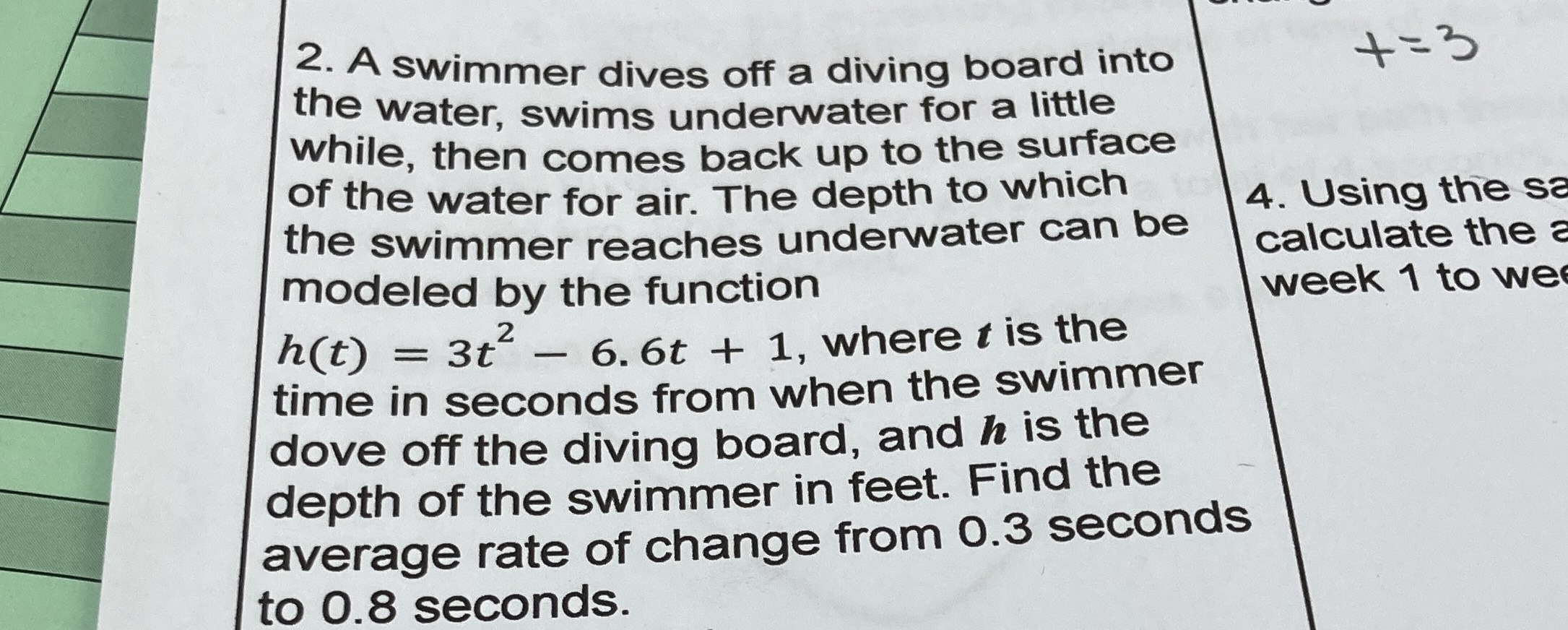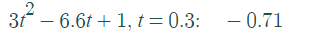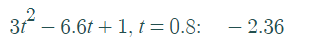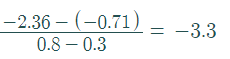### ¿Todavía tienes preguntas de matemáticas?

Pregunte a nuestros tutores expertos
Algebra
Pregunta2. A swimmer dives off a diving board into the water, swims underwater for a little while, then comes back up to the surface of the water for air. The depth to which the swimmer reaches underwater can be modeled by the function $$h ( t ) = 3 t ^ { 2 } - 6.6 t + 1$$ , where $$t$$ is the time in seconds from when the swimmer dove off the diving board, and $$h$$ is the depth of the swimmer in feet. Find the average rate of change from $$0.3$$ seconds to $$0.8$$ seconds.